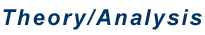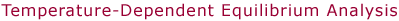Determination of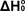from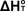Using Statistical Mechanics     The calculation of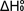from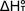can be made using the following equation, where the integral is over the different components of heat capacity: translational, rotational and vibrational (as well as electronic is some cases).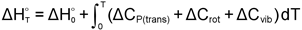However, we routinely use a somewhat more sophisticated method whereby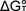is calculated as a function of temperature for direct comparison with our experimental data. In this approach, the various contributions to the entropy must also be included, as shown below andis then calculated as.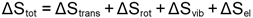A typical plot of experimentalvs. T is shown below along with the calculated fit. The difference between the straight line through the data points and the calculated curve at 0 K is exactly equal to the heat capacity correction given in the first equation above. By performing the analysis in this way, the parameters which affect the heat capacity corrections are tuned in order to reproduce the experimental entropy (roughly equal to the slope of thesevs. T plots).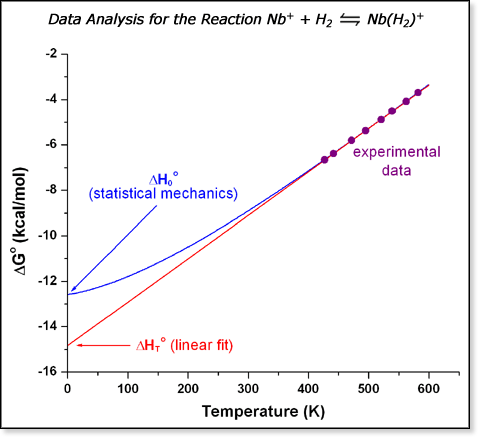Whether an analysis is carried out by simply calculating the heat capacity corrections or by modeling the entirevs. T curve, one needs various molecular parameters. These include the masses of the species involved (for translational terms), moments of inertia (for rotational terms), and vibrational frequencies (for vibrational terms). If there are low-lying excited electronic states that are thermally accessible, their relative energies are also needed for the electronic contribution. The masses are known - this is a mass-spectrometry-based method after all. Molecular modeling is usually used to determine the rotational constants and frequencies. Rotational constants are relatively easy to calculate from even a somewhat crude approximate structure. Vibrations are more difficult to calculate, but contribute much less to the final result. That is, the fitting procedure described here is relatively insensitive to errors in vibrational frequencies, and only frequencies of less than a few hundred wavenumbers contribute much to the heat capacity or entropy. There is a further term included to compensate for any instrumental mass discrimination that may exist. This accounts for any mass-dependent bias in the transmission through the final mass-selection stage of the experiment. Any mass discrimination, MD, will lead to an effective entropy correction of R·ln(MD).* Therefore, a reasonable set of parameters is chosen for a particular system, the fit to experiment is performed by varying MD as well as, and then the parameters are varied over a wide range (refitting MD andeach time) to get the resulting uncertainty in. For species with low-lying excited electronic states, the electronic terms are also added in, and differences in state multiplicities are also taken into account. For cases where H2 is used as a buffer gas, the full state counting approach to calculating the heat capacity and entropy are included due to the very large rotational temperature of dihydrogen. However, this is a rather small contribution to the final value of.     It is important to point out that, despite the seemingly large number of parameters that can be varied (vibrational frequencies, rotational constants, excited electronic state energies), this method gives well-determined values of. Sufficiently accurate values for the rotational constants are usually available from ab initio or molecular dynamics calculations, depending on the size of the system. Vibrational frequencies larger than a few hundred wavenumbers have little effect on the heat capacity and entropy, so usually only a small number of frequencies need to be varied. Also, excited electronic states with energies a few kcal/mol above the ground state also have little effect and such low-lying excited states are usually not present. Inaccuracies in these parameters generally lead to errors inof a few tenths of a kcal/mol.   *MD is defined as the ratio of transmission efficiency of product vs. reactant ions.